International
Tables for
Crystallography
Volume B
Reciprocal space
Edited by U. Shmueli

International Tables for Crystallography (2010). Vol. B, ch. 1.3, pp. 54-55   | 1 | 2 |

## Section 1.3.3.2.2. The Good (or prime factor) algorithm

G. Bricognea

aGlobal Phasing Ltd, Sheraton House, Suites 14–16, Castle Park, Cambridge CB3 0AX, England, and LURE, Bâtiment 209D, Université Paris-Sud, 91405 Orsay, France

| top | pdf |

#### 1.3.3.2.2.1. Ring structure on| top | pdf |

The setof congruence classes of integers modulo an integer N [see e.g. Apostol (1976), Chapter 5] inherits fromnot only the additive structure used in deriving the Cooley–Tukey factorization, but also a multiplicative structure in which the product of two congruence classes mod N is uniquely defined as the class of the ordinary product (in) of representatives of each class. The multiplication can be distributed over addition in the usual way, endowingwith the structure of a commutative ring.

If N is composite, the ringhas zero divisors. For example, let, letmod N, and letmod N: thenmod N. In the general case, a product of nonzero elements will be zero whenever these elements collect together all the factors of N. These circumstances give rise to a fundamental theorem in the theory of commutative rings, the Chinese Remainder Theorem (CRT), which will now be stated and proved [see Apostol (1976), Chapter 5; Schroeder (1986), Chapter 16].

#### 1.3.3.2.2.2. The Chinese remainder theorem

| top | pdf |

Letbe factored into a product of pairwise coprime integers, so that g.c.d.for. Then the system of congruence equationshas a unique solutionmod N. In other words, eachis associated in a one-to-one fashion to the d-tupleof its residue classes in.

The proof of the CRT goes as follows. Let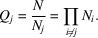Since g.c.d.there exist integersandsuch thatthen the integer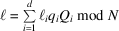is the solution. Indeed,because all terms withcontainas a factor; andby the defining relation for.

It may be noted that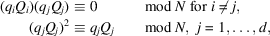so that theare mutually orthogonal idempotents in the ring, with properties formally similar to those of mutually orthogonal projectors onto subspaces in linear algebra. The analogy is exact, since by virtue of the CRT the ringmay be considered as the direct productvia the two mutually inverse mappings:

 (i)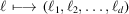bymodfor each j; (ii)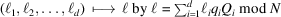.

The mapping defined by (ii)is sometimes called the `CRT reconstruction' offrom the.

These two mappings have the property of sending sums to sums and products to products, i.e: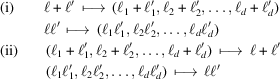(the last proof requires using the properties of the idempotents). This may be described formally by stating that the CRT establishes a ring isomorphism:#### 1.3.3.2.2.3. The prime factor algorithm

| top | pdf |

The CRT will now be used to factor the N-point DFT into a tensor product of d transforms, the jth of length.

Let the indices k andbe subjected to the following mappings:

 (i)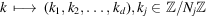, bymodfor each j, with reconstruction formula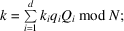(ii)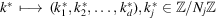, bymodfor each j, with reconstruction formula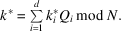Then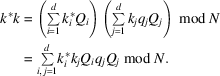Cross terms withvanish since they contain all the factors of N, hence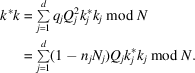Dividing by N, which may be written asfor each j, yieldsand henceTherefore, by the multiplicative property of,Letbe described by a one-dimensional arrayindexed by k. The index mapping (i)turns X into an element ofdescribed by a d-dimensional array; the latter may be transformed byinto a new array. Finally, the one-dimensional array of resultswill be obtained by reconstructingaccording to (ii).

The prime factor algorithm, like the Cooley–Tukey algorithm, reindexes a 1D transform to turn it into d separate transforms, but the use of coprime factors and CRT index mapping leads to the further gain that no twiddle factors need to be applied between the successive transforms (see Good, 1971). This makes up for the cost of the added complexity of the CRT index mapping.

The natural factorization of N for the prime factor algorithm is thus its factorization into prime powers:is then the tensor product of separate transforms (one for each prime power factor) whose results can be reassembled without twiddle factors. The separate factorswithin eachmust then be dealt with by another algorithm (e.g. Cooley–Tukey, which does require twiddle factors). Thus, the DFT on a prime number of points remains undecomposable.

### References

Apostol, T. M. (1976). Introduction to Analytic Number Theory. New York: Springer-Verlag.
Good, I. J. (1971). The relationship between two fast Fourier transforms. IEEE Trans. C-20, 310–317.
Schroeder, M. R. (1986). Number Theory in Science and Communication, 2nd ed. Berlin: Springer-Verlag.